# Converse Statement

Converse Statement

A statement that is of the form "If p then q" is a conditional statement. Here 'p' refers to 'hypotheses' and 'q' refers to 'conclusion'.

For example, "If Cliff is thirsty, then she drinks water."  This is a conditional statement. It is also called an implication. The converse statement is " If Cliff drinks water then she is thirsty".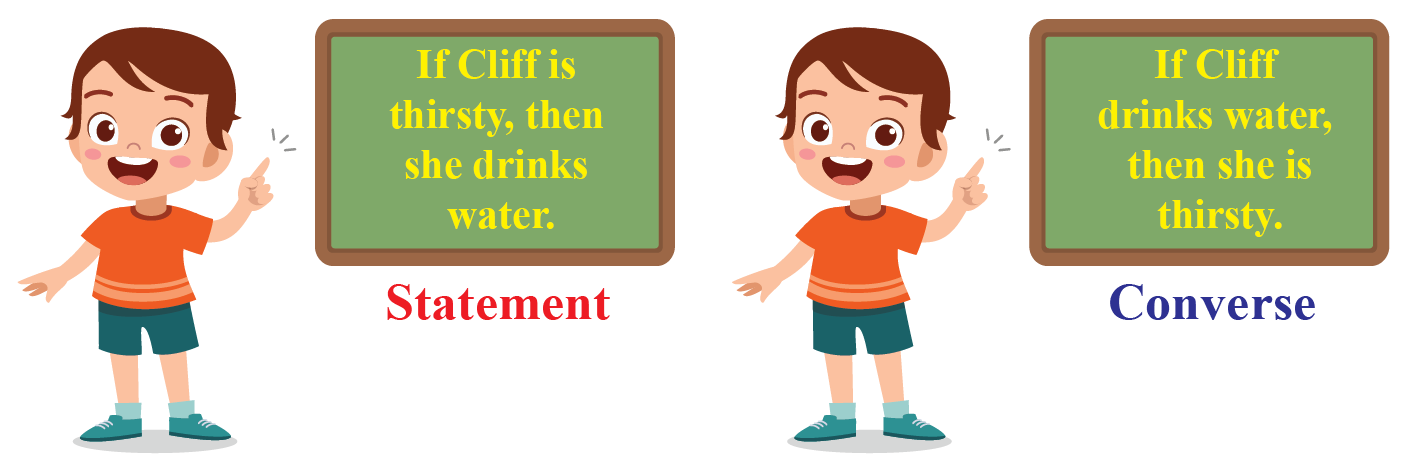A converse statement is the opposite of a conditional statement. It is to be noted that not always the converse of a conditional statement is true.

For example, in geometry, "If a closed shape has four sides then it is a square" is a conditional statement, The truthfulness of a converse statement depends on the truth of hypotheses of the conditional statement.

In this mini-lesson, we will learn about the converse statement, how inverse and contrapositive are obtained from a conditional statement, converse statement definition, converse statement geometry, and converse statement symbol.

## Lesson Plan

 1 What Is Converse Statement? 2 Important Notes on Converse Statement 3 Thinking out of the Box! 4 Solved Examples on Converse Statement 5 Interactive Questions on Converse Statement

## What Is Converse Statement?

### Definition

A statement obtained by reversing the hypothesis and conclusion of a conditional statement is called a converse statement.

### Explanation

Let us understand the terms "hypothesis" and "conclusion."

A statement which is of the form of "if p then q" is a conditional statement, where 'p' is called hypothesis and 'q' is called the conclusion.

A converse statement is gotten by exchanging the positions of 'p' and 'q' in the given condition.

 if \begin{align} p \rightarrow q,\end{align} then, \begin{align} q \rightarrow p\end{align}

For example,

"If Cliff is thirsty, then she drinks water" is a condition.

The converse statement is "If Cliff drinks water, then she is thirsty."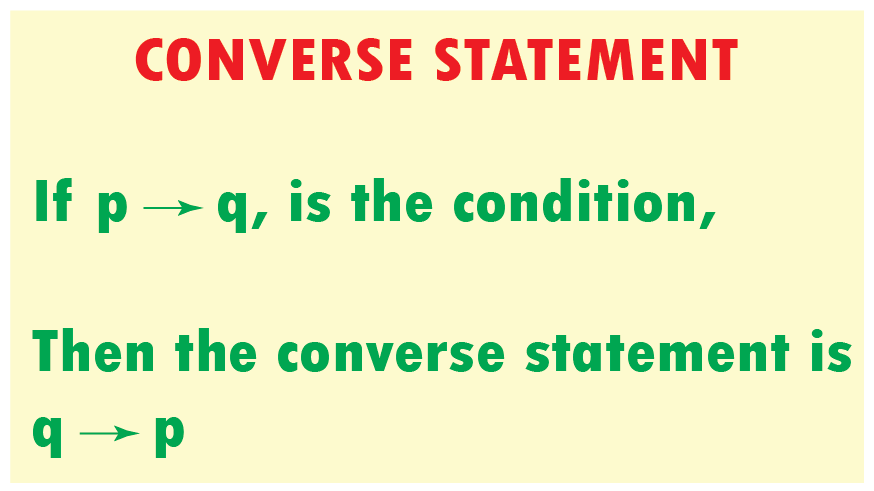The hypothesis 'p' and conclusion 'q' interchange their places in a converse statement.

## What Is Inverse Statement?

### Definition

A statement obtained by negating the hypothesis and conclusion of a conditional statement.

### Explanation

An inverse statement changes the "if p then q" statement to the form of  "if not p then not q."

 if \begin{align} p \rightarrow q,\end{align} then, \begin{align} \sim{p} \rightarrow \sim{q}\end{align}

For example,

"If John has time, then he works out in the gym."

The inverse statement is "If John does not have time, then he does not work out in the gym."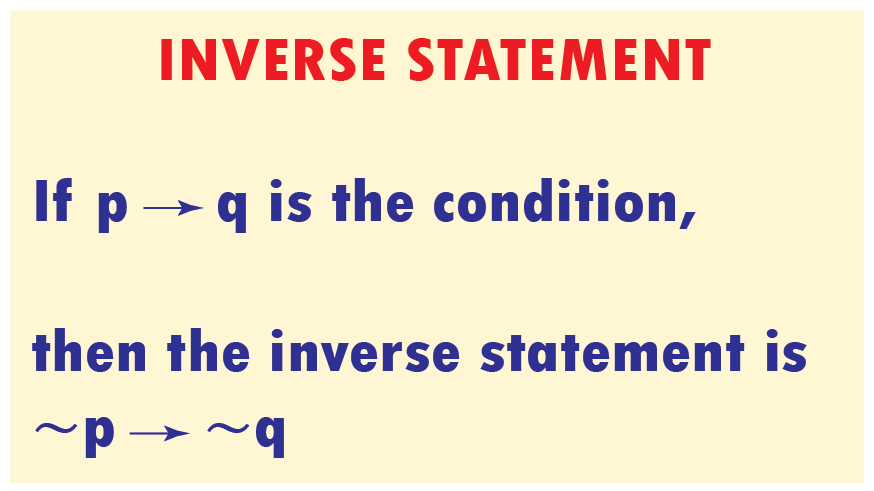## What Is Contrapositive Statement?

### Definition

A statement obtained by exchanging the hypothesis and conclusion of an inverse statement.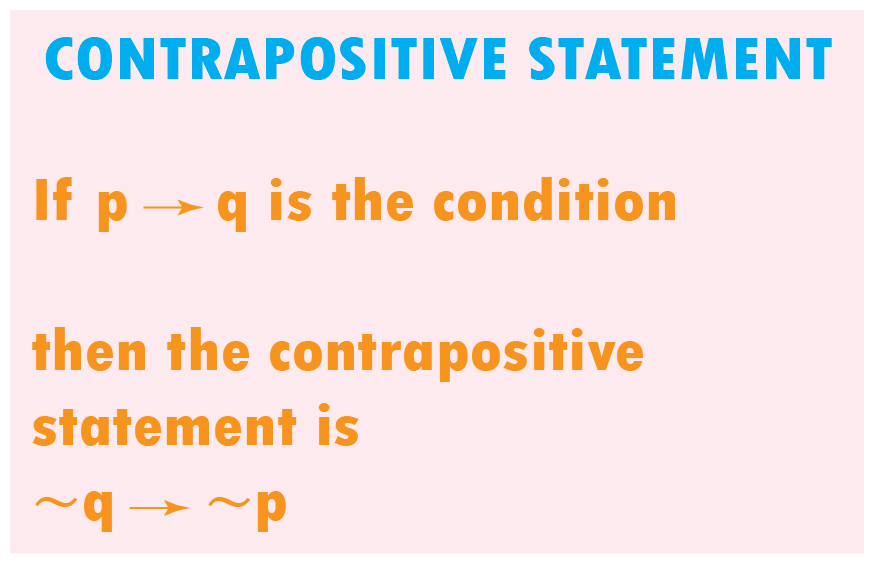### Explanation

A contrapositive statement changes "if not p then not q" to "if not q to then, not p."

• The converse of the conditional statement is “If Q then P.”
• The contrapositive of the conditional statement is “If not Q then not P.”
• The inverse of the conditional statement is “If not P then not Q.”
 if \begin{align} p \rightarrow q,\end{align} then, \begin{align} \sim{q} \rightarrow \sim{p}\end{align}

For example,

If it is a holiday, then I will wake up late. - Conditional statement

If it is not a holiday, then I will not wake up late. - Inverse statement

If I am not waking up late, then it is not a holiday. - Contrapositive statement

## What Is a Conditional Statement?

### Definition

A conditional statement is a statement in the form of "if p then q," where 'p' and 'q' are called a hypothesis and conclusion.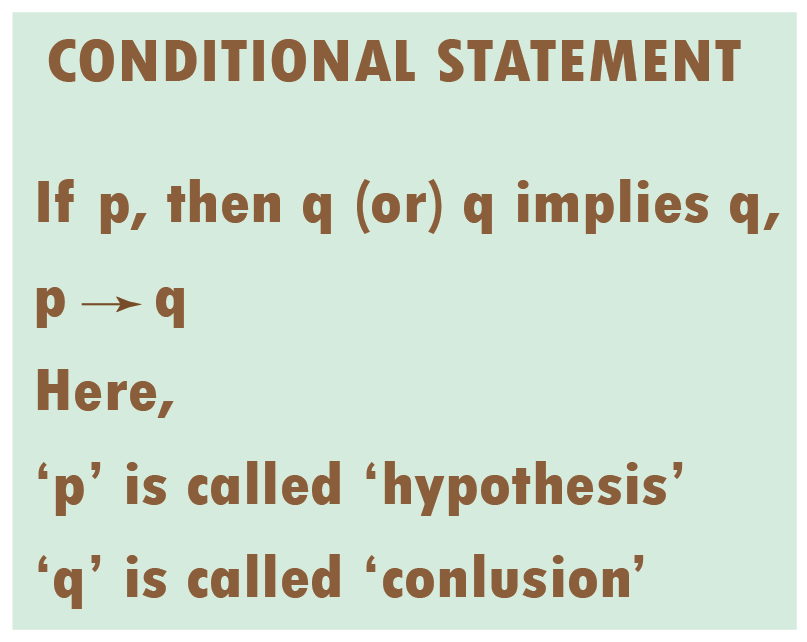A conditional statement defines that if the hypothesis is true then the conclusion is true.

For example,

"If we have to to travel for a long distance, then we have to take a taxi" is a conditional statement.

## Converse of a Conditional Statement

To get the converse of a conditional statement, interchange the places of hypothesis and conclusion.

 if \begin{align} p \rightarrow q,\end{align} then, \begin{align} q \rightarrow p\end{align}

For example,

If you eat a lot of vegetables, then you will be healthy. - Conditional statement

If you are healthy, then you eat a lot of vegetables. - Converse of Conditional statement

## Inverse of Conditional Statement

To get the inverse of a conditional statement, we negate both the hypothesis and conclusion.

 if \begin{align} p \rightarrow q,\end{align} then, \begin{align} \sim{p} \rightarrow \sim{q}\end{align}

For example,

If you read books, then you will gain knowledge. - Conditional statement

If you do not read books, then you will not gain knowledge. -Inverse of conditional statement

## Contrapositive of Conditional Statement

To get the contrapositive of a conditional statement, we negate the hypothesis and conclusion and exchange their position.

 if \begin{align} p \rightarrow q,\end{align} then, \begin{align} \sim{q} \rightarrow \sim{p}\end{align}

For example,

Emily's dad watches a movie if he has time. - Conditional statement

If Emily's dad does not have time, then he does not watch a movie. - Contrapositive of a conditional statement

More Important Topics
More Important Topics
Learn from the best math teachers and top your exams

• Live one on one classroom and doubt clearing
• Practice worksheets in and after class for conceptual clarity
• Personalized curriculum to keep up with school